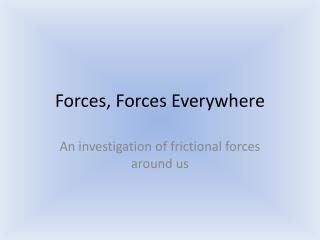Download PresentationForces, Forces Everywhere

# Forces, Forces Everywhere

Télécharger la présentation## Forces, Forces Everywhere

- - - - - - - - - - - - - - - - - - - - - - - - - - - E N D - - - - - - - - - - - - - - - - - - - - - - - - - - -
##### Presentation Transcript

1. Forces, Forces Everywhere An investigation of frictional forces around us

2. Forces • Newton’s 2nd law: F=m*a • Weight is an example of Force • Gravity is an example of Acceleration • Forces can be described by the direction in which the acceleration acts • Forces acting on surfaces • Normal Force

3. “Nonconservative” Forces • Friction • Proportional to the normal force • Exists for motionless and moving objects. • Ff=μN • Drag • Proportional to velocity of fluid or object • Fd=(½)ρv2CdA • Density, velocity, drag coefficient, area

4. Purpose • We will experiment with friction by examining the force needed to move two surfaces past each other, and analyze the force-position data to calculate frictional coefficient(s).

5. Hypothesis **Think about these questions** Will the roughness of the wheels and sliding surface (floor) have an effect on the force required to move the car? How are we comparing surface roughness?